## What is stellar magnitude?

Brightest stars to the eye are 1st magnitude, and dimmest stars to the naked eye are 6th magnitude. How the magnitude scale works in astronomy and why it’s useful.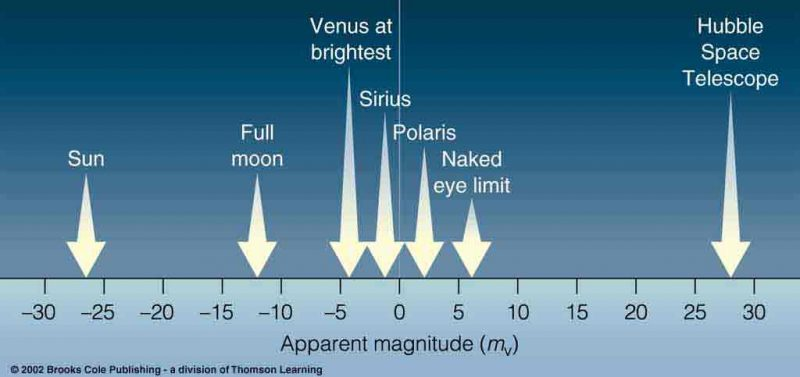Sometimes you’ll read how bright a star or planet appears from Earth at a particular magnitude. The word magnitude in astronomy, unless stated otherwise, usually refers to a celestial object’s apparent brightness or apparent visual magnitude. The intrinsic brightness of stars, on the other hand, is called luminosity or absolute magnitude. For the remainder of this post, we’ll be using the word magnitude to talk about a star’s apparent visual magnitude.

The magnitude scale dates back to the ancient astronomers Hipparchus and Ptolemy, whose star catalogs listed stars by their magnitudes.

According to this ancient scale, the brightest stars in our sky are 1st magnitude, and the very dimmest stars to the eye alone are 6th magnitude. A 2nd-magnitude star is still modesty bright but fainter than a 1st-magnitude star, and a 5th-magnitude star is still pretty faint but brighter than a 6th-magnitude star.

This system remains intact to this day, though with some modification.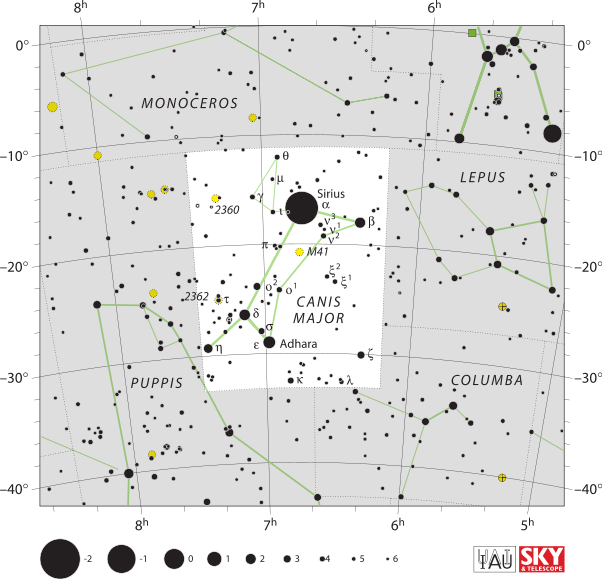People often find the magnitude system confusing because the brightest stars have negative magnitudes. For instance, the star Sirius, the brightest star of the nighttime sky, has an apparent magnitude of -1.44.

In the spirit of Hipparchus and Ptolemy, the modern-day charts of the constellation Canis Major above and the constellation Virgo below scale stars by magnitude.

Take the star Spica, the sole bright star in Virgo. It serves as a prime example of a 1st-magnitude star.

In other words, although Spica’s magnitude is slightly variable, its magnitude almost exactly equals 1.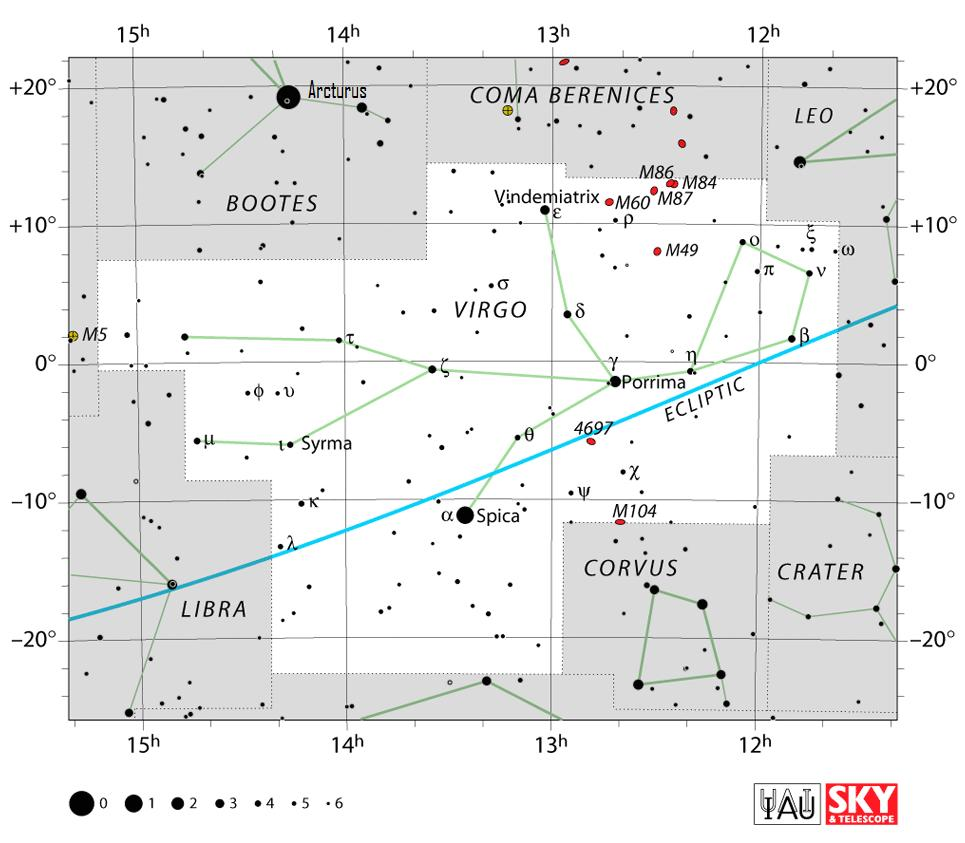Notice the magnitude scale at the lower left corner of this star chart and the one above. Sky charts via the International Astronomical Union (IAU). Click here for sky charts of all 88 constellations.

Of course, most stars aren’t like Spica in that they don’t fall so precisely at a whole number on the magnitude scale. That’s why – for astronomers – any star with a magnitude between 0.50 and 1.50 is considered to be of 1st-magnitude brightness.

Consider the 1st-magnitude star Aldebaran, which has an apparent magnitude of 0.87. Meanwhile, the 1st-magnitude star Regulus has a magnitude of 1.36. Both are considered 1st magnitude stars – among the sky’s brightest stars – although their brightnesses are not exactly equal.

Okay, let’s talk about some astronomical shorthand. In astronomy, the intrinsic or true brightness of a star – sometimes called its absolute magnitude is represented by a capital letter M. Meanwhile, apparent magnitude – or how bright a star appears from Earth – is presented by a lower case letter m.

This system of numbering for apparent magnitude confuses some people. Just remember, the dimmer the star, the higher the magnitude number. Regulus (m = 1.36) is actually fainter than Spica (m = 1), yet Aldebaran (m = 0.87) is brighter than Spica.

The magnitude scale is much like golf in that the lower number means a greater brightness on the magnitude scale and a better score in golf.

Loosely speaking, the 21 stars that are brighter than magnitude 1.50 are called 1st-magnitude stars.

However, the 0-magnitude star Vega (m = 0.00) is actually one magnitude brighter than Spica (m = 1.00), and the star Sirius with a negative magnitude (m = -1.44) is nearly two and one-half magnitudes brighter than Spica.

One magnitude corresponds to a brightness factor of 2.512 times

Modern astronomy has added precision to the magnitude scale. A difference of 5 magnitudes corresponds to a brightness factor of a hundredfold. In other words, a 1st-magnitude star is 100 times brighter than a 6th-magnitude star – or conversely, a 6th-magnitude star is 100 times dimmer than a 1st-magnitude star. The fifth root of 100 approximately equals 2.512, so a difference of one magnitude corresponds to a brightness factor of about 2.512 times.

1m: brightness factor of 2.512
2m: brightness factor of 2.512 x 2.512 = 6.31
3m: brightness factor of 2.512 x 2.512 x 2.512 = 15.84
4m: brightness factor of 2.512 x 2.512 x 2.512 x 2.512 = 39.81
5m: brightness factor of 2.512 x 2.512 x 2.512 x 2.512 x 2.512 = 100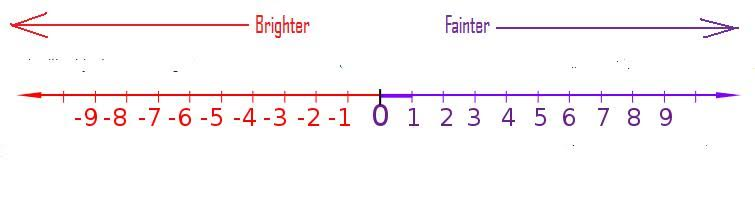A higher positive number means a fainter celestial object; whereas a higher negative number means a brighter celestial object. For instance, Venus at its brightest has a magnitude of -4.6 and the faintest star visible to the naked eye has a magnitude of +6.0.

Extending the magnitude scale

However, there’s a far larger range of brightness than just 5 magnitudes (brightness factor of one hundredfold) in our sky. The sun, moon, plus the planets Venus and Jupiter are much, much brighter than 1st-magnitude; and telescopes let us see stars that are millions of times fainter than 6th-magnitude.

Nowadays, the magnitude system includes not just stars but also the sun, moon, planets, asteroids and comets within the solar system, and star clusters and galaxies that reside outside the solar system. Astronomers even list the magnitudes of man-made satellites circling our planet.

Because a difference of 5 magnitudes corresponds to a brightness factor of 100 times, then a difference of 10 magnitudes corresponds to a brightness factor of 10,000 times (100 x 100 = 10,000). In addition, a difference of 15 magnitudes corresponds to a brightness factor of 1,000,000 times, and a difference of 20 magnitudes corresponds to a brightness factor of 100,000,000 times.

10m = 100 x 100 = brightness factor of 10,000 times
15m = 100 x 100 x 100 = brightness factor of 1,000,000 times
20m = 100 x 100 x 100 x 100 = brightness factor of 100,000,000 times

Click on Skylive.com to know the current magnitudes of the sun, moon and planets (plus the brighter comets and asteroids).

Magnitudes of Celestial Objects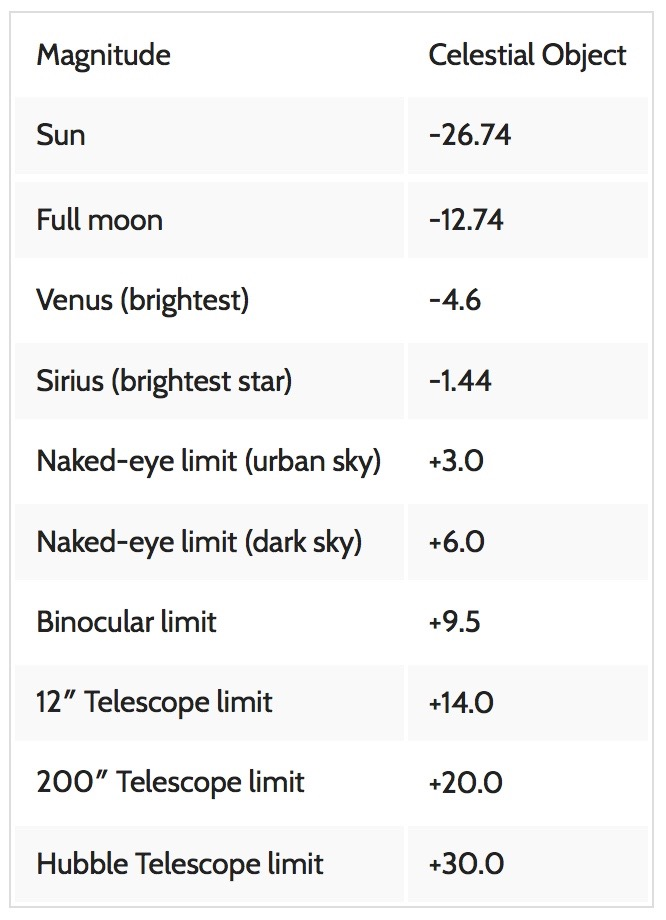How much brighter is the sun than the full moon?

Looking at the table above, we find the magnitude of the sun at -26.74 and the full moon at -12.74. Those numbers may be abstract to the point of meaningless for many of us, but let’s see if we can bring this arcane magnitude system down to Earth. First of all, we find that the magnitude difference between the sun and moon equals 14 magnitudes: -12.74 -(-26.74) = -12.74 + 26.74 = 14.00.

Magnitude difference of sun and full moon: -12.74 -(-26.74) = -12.74 + 26.74 = 14.00

Or if you prefer:

Magnitude difference of sun and full moon: -26.74 -(-12.74) = -26.74 + 12.74 = -14.00

We can divide this magnitude difference between the sun and moon into 10m and 4m. Looking at our chart above, we see 10m = a brightness factor of 10,000 and 4m = a brightness factor of 39.81. We then multiply 10,000 by 39.81 to find that the sun is nearly 400,000 times brighter than the full moon.

Brightness variation of sun and full moon: 10,000 x 39.81 = 398,100 times brighter than the full moon.

Bottom line: The stellar magnitude system devised by ancients was much less confusing when it only applied to those stars visible to the unaided eye. The brightest stars were 1st-magnitude and the faintest stars were 6th-magnitude. However, modern astronomy has expanded the magnitude scale to include brighter celestial objects (such as the sun, moon and Venus) and in the other direction to telescopic objects that lie beyond the limit of the naked eye. Therefore, the brightest celestial objects have the highest negative numbers while the faintest have the highest positive numbers.

### Bruce McClure